# Solving the 4x4x4

back to Rubik's Cube

 7.1 Solve the first center 7.1.1a Construct a matching 2x1 block 7.1.1bF (D d)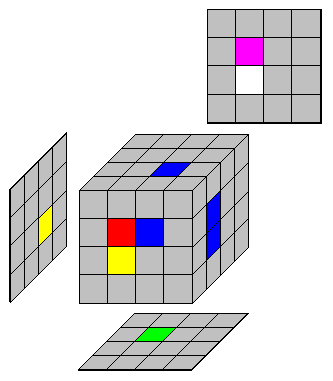7.1.2a Pair up the remaining center cubies 7.1.2bU2 (R r)7.1.3a Join the blocks together 7.1.3bR' (F' f')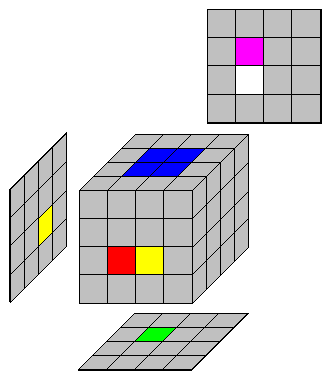7.2 Solve remaining centers 7.2.1a Swap Ufr with Ful 7.2.1b(R' r') F (R r)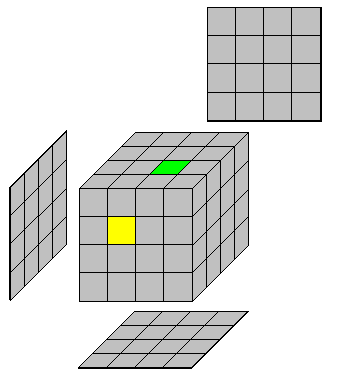7.3 Solve remaining centers 7.2.1a Swap Ufr with FulF(R' r') F (R r)7.4 Swapping opposite centers 7.4.1a Swap the Up and Bottom centers 7.4.1b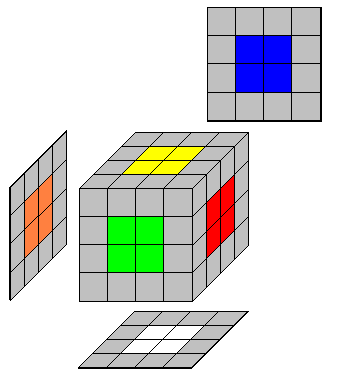(R2 r2) U2 D2 (R2 r2)7.5 Swapping adjacent centers 7.5.1a Swap Front and Right centers 7.5.1b(U' u') R2 (U u) (D d) R2 (D' d')7.6 Solve the first four double edges (dedges) 7.6.1 The other edge is in the top layer on the left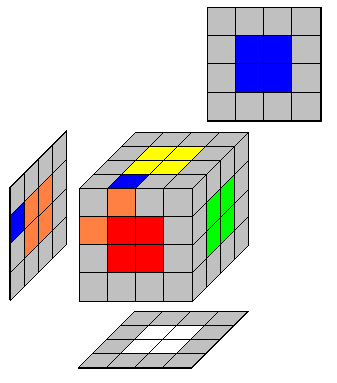R U' R7.6.2 The other edge is in the top layer on the rightU' F' U F7.6.3 The other edge is in the bottom layer on the left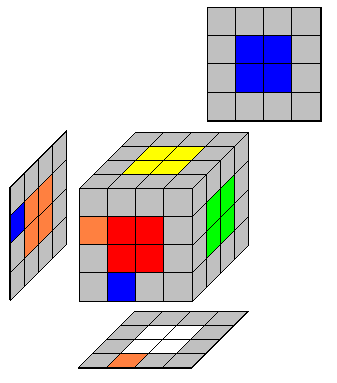D F D' F'7.6.4 The other edge is in the bottom layer on the rightR' D R7.6.5 The other edge is in a middle layerR U R'7.7 Pairing up the dedge(D' d')7.8 Storing the dedge in the U layerL' U L (D d)7.9 The dedge is in the middle layer 7.6.1 The other edge is in the top layer on the leftR U' R'7.10 Solve the final four dedges 7.10.1a Other edge is in the FL position Do nothing - the dedge is solved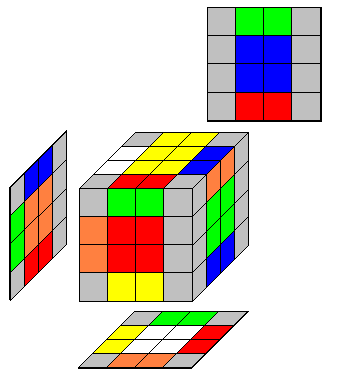7.10.2a Other edge is in the FR position Go to 7.11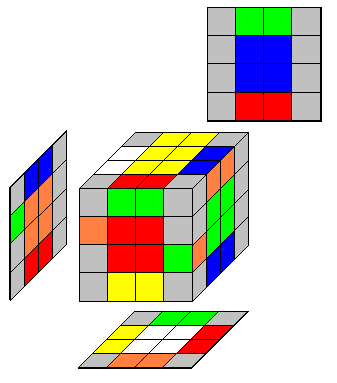7.10.3a Other edge is in the BR positionR2 7.10.3a Other edge is in the BL position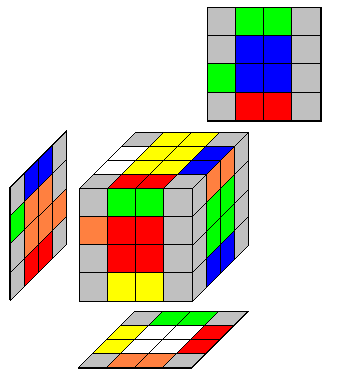B2 R2

 7.11 The final four dedges: Flipping the edge pair 7.11.1 The other edge is in the dFR position goto 7.127.11.2 The other edge is in the uFR position Then goto 7.12R U R' F R' F' R

 7.12 The final four dedges: Final step 7.12.1(D d) R U R' F R' F' R (D' d')7.13 Fixing orientation parity 7.13.1 The FU edge needs to be flipped - leaves corners unchanged Use one of thesel2 B2 l U2 l U2 x' U2 l U2 l' U2 l U2 l2 U2 x r2 B2 r' U2 r' U2 x' U2 r' U2 r U2 r' U2 r2 U2 x r2 B2 U2 l U2 r' U2 r U2 F2 r F2 l' B2 r2 7.13.2 The FU edge needs to be flipped - corners are altered(L2 l2) B2 (L l) U2 (L l) U2 x' U2 (L l) U2 (L' l') U2 (L l) U2 (L2 l2) U2 x (R2 r2) B2 (R' r') U2 (R' r') U2 x' U2 (R' r') U2 (R r) U2 (R' r') U2 (R2 r2) U2 x (R2 r2) B2 U2 (L l) U2 (R' r') U2 (R r) U2 F2 (R r) F2 (L l') B2 (R2 r2)